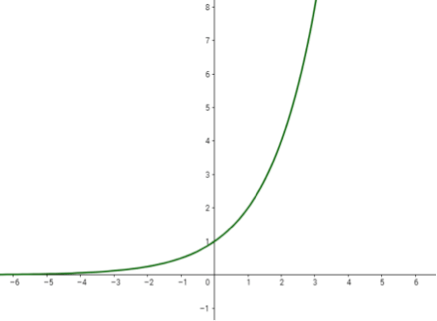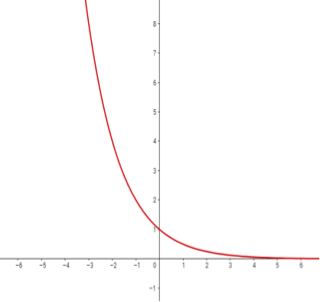# Graphs of Growth and Decay

 Exponential Growth$$f(x)=2^x$$ Exponential Decay$$f(x)= \left( \Large \frac{1}{2} \right) ^x$$

The graphs above are examples of an Exponential Growth function and an Exponential Decay function.  What do you notice about the two graphs? How are they alike/different?

Click on the link for the Exponential Graphs by Desmos and explore the changes made by  sliding the slider for various values of $$a$$ and $$b$$. Describe in words the effect of changing $$a$$ and $$b$$ on the shape of the graph.